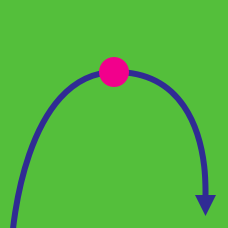Calculus

# Curve Sketching

Let $P$ and $Q$ be the two inflection points of the curve $\displaystyle y=e^{-5x^2}.$ What is the area of the triangle $OPQ,$ where $O$ is the origin?

If $\displaystyle{f(x)=\frac{1}{x^2+9}},$ the interval on which the curve $y=f(x)$ is concave down can be expressed as $a What is the value of $ab?$

Let $\displaystyle{f(x)=8+\frac{a}{x}+\frac{b}{x^2},}$ where $a$ and $b$ are constants. If the point $(-1,0)$ is the inflection point of the curve $y=f(x),$ what is $a+b?$

If $f(x) = x^{1/3}(x + 26)$, the interval on which the curve $y = f(x)$ is concave down can be expressed as $a < x < b$. What is the value of $a + b$?

Let $y=(ax^2+30)e^x$ be the equation of a curve, where $a$ is a constant. If $A$ and $B$ are two distinct arbitrary points on the curve and the arc between the two points always lies below the line connecting the two points, what is the range of $a?$

×